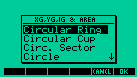Inertia is a program that calculates XG,YG,IG and area of 18 plain bodies. It calculates XG,YG,IG and area of the Circular Sector, Quarter of Circle, Circular Cup, Square, Circular Ring, Circle,Triangle, Ellipsis, Lozangue, Rectangle with Hole, Parallelogram, Square with Hole, Plygon of n sides, Half Circle, Half Ellipsis Half Circle Upsidown and Double TScreenshot of INERTIA